# GED Math : Statistics

## Example Questions

### Example Question #15 : Median

Identify the median: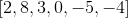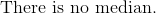Explanation:

Reorder the numbers from least to greatest.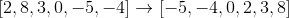For an even number of values in a set of data, the median is the average of the central two numbers.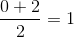The answer is:### Example Question #16 : Median

Give the median of the following test scores:

12, 34, 22, 28, 22, 28, 19, 20, 19, 22, 29, 35, 23Explanation:

There are thirteen test scores, an odd number, so the median of the test scores is the score which, after the scores are ordered, appears in the middle. Order the scores from greatest to least:

12, 19, 19, 20, 22, 22, 22, 23, 28, 28, 29, 34, 35

As there are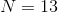scores, we are seeking out the score that ranks at number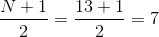That is, the median is the seventh-highest score. This score can be seen to be 22.

### Example Question #111 : Statistics

Give the median of the following test scores:

12, 34, 22, 28, 22, 28, 19, 20, 19, 22, 29, 50, 35, 23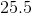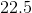Explanation:

There are fourteen test scores, an even number, so the median of the test scores is the arithmetic mean of the two scores which, after the scores are ordered, appear in the middle. Order the scores from greatest to least:

12, 19, 19, 20, 22, 22, 22, 23, 28, 28, 29, 34, 35, 50

As there are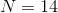scores, we are seeking out the score that ranks at number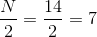from the top, and number 7 from the bottom.

That is, the median is the arithmetic mean of the seventh-highest and seventh-lowest scores. These scores can be seen to be 22 and 23, so the median of the scores is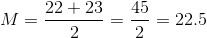### Example Question #111 : Statistics

Identify the median: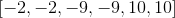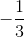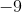Explanation:

Reorder the numbers from least to greatest.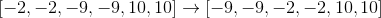For an even amount of numbers given, the median is the average of the central two numbers.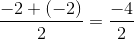The answer is:### Example Question #112 : Statistics

Use the following data set of student test scores to answer the question: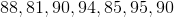Find the median.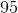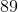Explanation:

To find the median of a data set, we will first arrange the numbers in ascending order. Then, we will find the number in the middle of the set.

So, given the data setwe will arrange the numbers in ascending order (from smallest to largest). We get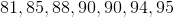Now, we will find the number in the center. We get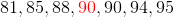We can see that it is 90.

Therefore, the median of the data set is 90.

### Example Question #113 : Statistics

Use the following data set to answer the question: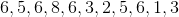Find the median.Explanation:

To find the median of a data set, we will first arrange the numbers in ascending order. Then, we will find the number in the middle of the set.

So, given the data setwe will first arrange the numbers in ascending order (from smallest to largest). So, we get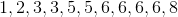Now, we will find the number in the middle.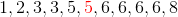We can see that it is 5.

Therefore, the median of the data set is 5.

### Example Question #21 : Median

Determine the median of the numbers: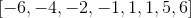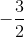Explanation:

The numbers are already arranged in chronological order.

For an even set of numbers, the median is the average of the central two numbers.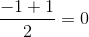The answer is:### Example Question #1931 : Ged Math

Use the following data set to answer the question: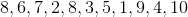Find the median.Explanation:

To find the median of a data set, we will first arrange the numbers in ascending order. Then, we will find the number in the middle of the set.

So, given the data setwe will first arrange them in ascending order (from smallest to largest). We get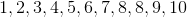Now, we will find the number in the middle.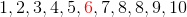We can see that it is 6.

Therefore, the median of the data set is 6.

### Example Question #23 : Median

Find the median: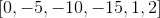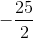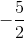Explanation:

Rearrange the numbers from least to greatest.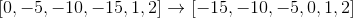For an even set of numbers, the median is the average of the two central numbers.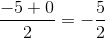The answer is:### Example Question #114 : Statistics

Determine the median of the following numbers: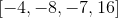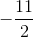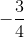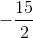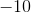Explanation:

Rearrange the numbers from least to greatest.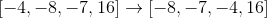The median of the data set is the average of the two central numbers, since there is an even amount of numbers provided.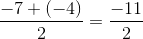The answer is:### All GED Math Resources